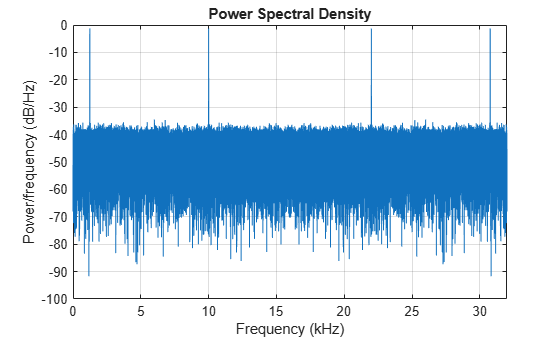# dspdata.psd

Power spectral density

## Syntax

```Hpsd = dspdata.psd(Data) Hpsd = dspdata.psd(Data,Frequencies) Hpsd = dspdata.psd(...,'Fs',Fs) Hpsd = dspdata.psd(...,'SpectrumType',SpectrumType) Hpsd = dspdata.psd(...,'CenterDC',flag) ```

## Description

The power spectral density (PSD) is intended for continuous spectra. The integral of the PSD over a given frequency band computes the average power in the signal over that frequency band. In contrast to the mean-squared spectrum, the peaks in this spectra do not reflect the power at a given frequency. See the `avgpower` method of `dspdata` for more information.

A one-sided PSD contains the total power of the signal in the frequency interval from DC to half of the Nyquist rate. A two-sided PSD contains the total power in the frequency interval from DC to the Nyquist rate.

`Hpsd = dspdata.psd(Data)` uses the power spectral density data contained in `Data`, which can be in the form of a vector or a matrix, where each column is a separate set of data. Default values for other properties of the object are shown below:

Property

Default Value

Description

`Name`

`'Power Spectral Density'`

`Frequencies`

`[]`

type `double`

Vector of frequencies at which the power spectral density is evaluated. The range of this vector depends on the `SpectrumType` value. For one-sided, the default range is [0, π) or [0, `Fs`/2) for odd length, and [0, π] or [0, `Fs`/2] for even length, if `Fs` is specified. For two-sided, it is [0, 2pi) or [0, `Fs`).

If you do not specify `Frequencies`, a default vector is created. If one-sided is selected, then the whole number of FFT points (nFFT) for this vector is assumed to be even.

If `onesided` is selected and you specify `Frequencies`, the last frequency point is compared to the next-to-last point and to π (or `Fs`/2, if `Fs` is specified). If the last point is closer to π (or `Fs`/2) than it is to the previous point, nFFT is assumed to be even. If it is closer to the previous point, nFFT is assumed to be odd.

The length of the `Frequencies` vector must match the length of the columns of `Data`.

`Fs`

`'Normalized'`

Sampling frequency, which is `'Normalized'` if `NormalizedFrequency` is `true`. If `NormalizedFrequency` is `false` `Fs` defaults to `1`.

`SpectrumType`

`'Onesided'`

Nyquist interval over which the power spectral density is calculated. Valid values are `'Onesided'` and `'Twosided'`. A one-sided PSD contains the total signal power in half the Nyquist interval. See the `onesided` and `twosided` methods in `dspdata` for information on changing this property.

The range for half the Nyquist interval is [0 pi) or [0 pi] depending on the number of FFT points. For the whole Nyquist interval, the range is [0 2pi).

`NormalizedFrequency`

`true`

Whether the frequency is normalized (`true`) or not (`false`). This property is set automatically at construction time based on `Fs`. If `Fs` is specified, `NormalizedFrequency` is set to `false`. See the `normalizefreq` method in `dspdata` for information on changing this property.

`Hpsd = dspdata.psd(Data,Frequencies)` uses the power spectral density estimation data contained in `Data` and `Frequencies` vectors.

`Hpsd = dspdata.psd(...,'Fs',Fs)` uses the sampling frequency `Fs`. Specifying `Fs` uses a default set of linear frequencies (in `Hz`) based on `Fs` and sets `NormalizedFrequency` to `false`.

`Hpsd = dspdata.psd(...,'SpectrumType',SpectrumType)` specifies the interval over which the power spectral density is calculated. For data that ranges from [0 π) or [0 π], set the `SpectrumType` to `onesided`; for data that ranges from [0 2π), set the `SpectrumType` to `twosided`.

`Hpsd = dspdata.psd(...,'CenterDC',flag)` uses the value of `flag` to indicate whether the zero-frequency (DC) component is centered. If `flag` is `true`, it indicates that the DC component is in the center of the two-sided spectrum. Set the `flag` to `false` if the DC component is on the left edge of the spectrum.

### Methods

Methods provide ways of performing functions directly on your `dspdata` object. You can apply a method directly on the variable you assigned to your `dspdata.psd` object. You can use the following methods with a `dspdata.psd` object.

• `avgpower`

• `centerdc`

• `normalizefreq`

• `onesided`

• `plot`

• `twosided`

For example, to normalize the frequency and set the `NormalizedFrequency` parameter to true, use

```Hpsd = normalizefreq(Hpsd) ```

For detailed information on using the methods and plotting the spectrum, see the `dspdata` reference page.

## Examples

collapse all

Estimate the one-sided power spectral density of a noisy sinusoidal signal with two frequency components.

```Fs = 32e3; t = 0:1/Fs:2.96; x = cos(2*pi*t*1.24e3)+ cos(2*pi*t*10e3)+ randn(size(t)); nfft = 2^nextpow2(length(x)); Pxx = abs(fft(x,nfft)).^2/length(x)/Fs;```

Store the spectrum in a PSD data object and plot the result.

```Hpsd = dspdata.psd(Pxx(1:length(Pxx)/2),'Fs',Fs); plot(Hpsd)```Create a two-sided spectrum and plot it.

```Hpsd = dspdata.psd(Pxx,'Fs',Fs,'SpectrumType','twosided'); plot(Hpsd)```